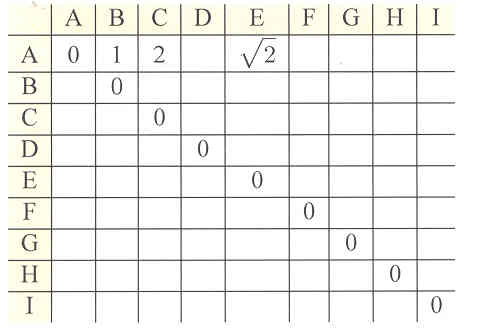# Individual Research Projects Section 5.5

### Project 5.8

You may have seen a geoboard, which is simply a board with pegs. Two simple geoboards with the pegs labeled A, B, C, ….

Distance on a Geoboard

Suppose that the horizontal and vertical distance between the pegs is 1 inch. We can then calculate the distance from A to D to be the square root of two (by the Pythagorean theorem). If we adopt the agreement that the distance from any point to itself is 0, we can find the distance from any peg to any other peg.Use the 3 x 3 peg board shown here to fill in the entries in the following table. Note that some of the entries have been filled in for you.

### Project 5.9

Prove that the positive square root of 2 is not rational.

### Project 5.10 Historical Quest

Write a paper or prepare an exhibit illustrating the Pythagorean theorem. Here are some questions you might consider:
What is the history of the Pythagorean theorem?
What are some unusual proofs of the Pythagorean theorem?
What are some of the unusual relationships that exist among Pythagorean numbers?
What models can be made to visualize the Pythagorean theorem?